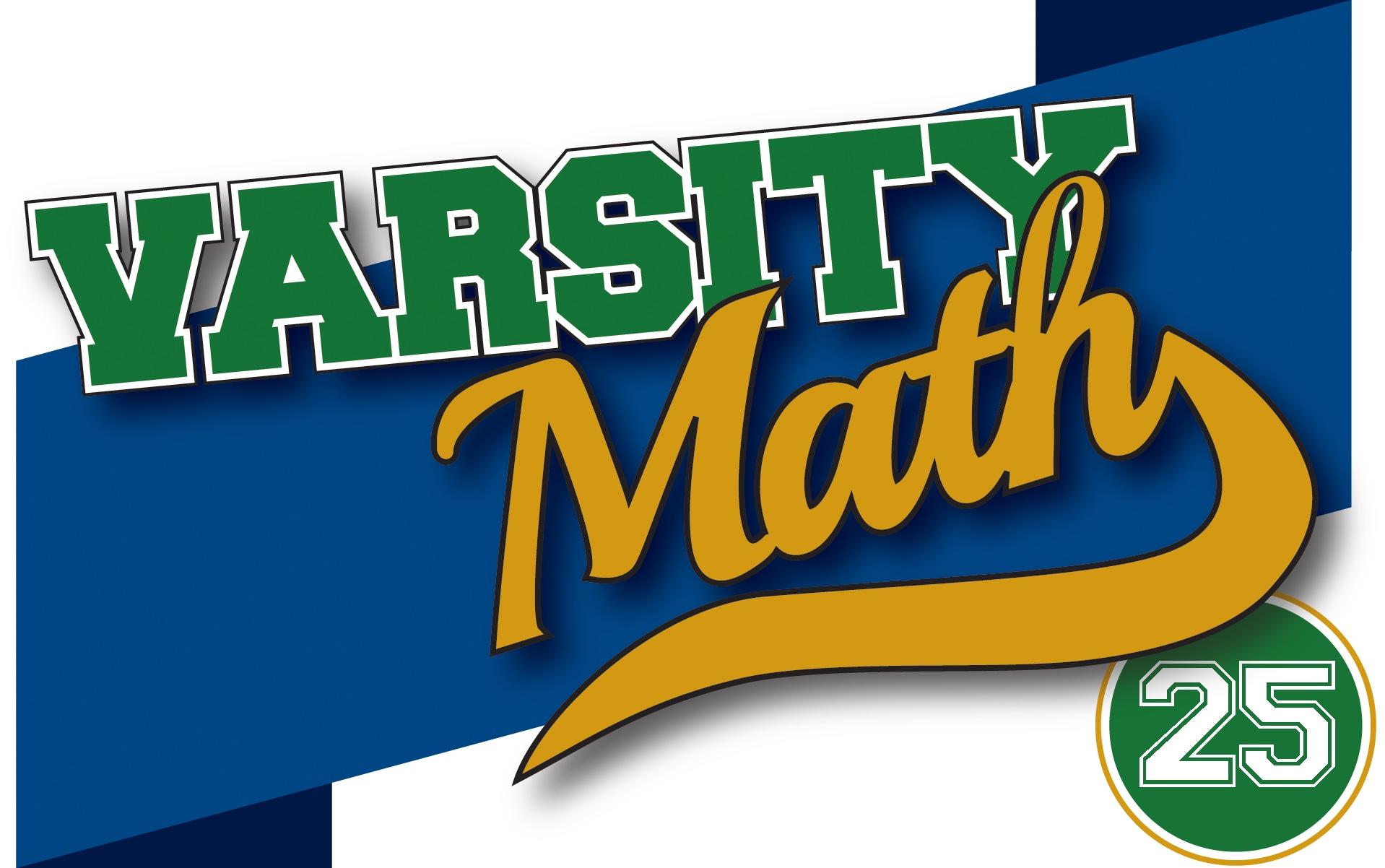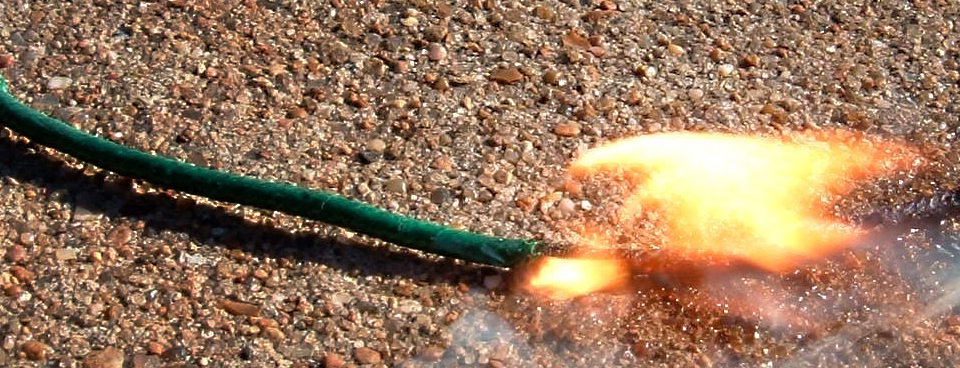This week, the team has a couple of new twists on some classic math puzzles.

##### Time to Burn

You have two 60-minute fuses — pieces of specially-treated string that take exactly 60 minutes to burn from one end to the other. However, the burn rate of these fuses may be quite irregular; any given centimeter of the fuses may take more or less time to burn than any other. You just know that the total burn time of each fuse is 60 minutes; the two fuses may be different physical lengths and the burn rates of the two of them will not necessarily follow the same pattern along their lengths. Assume that you have an unlimited supply of matches, a pair of scissors, and a damper that lets you put out any fuse at any time.

How do you measure 80 minutes, within a few seconds of accuracy, using only these two fuses?

[asciimathsf]

### Durable Digits

The reversal of a number is produced by reversing the order of its digits and deleting any leading zeros to form a new number. Quinn starts with a four-digit number and subtracts its reversal, which happens to be a smaller number. Quinn then takes the result and adds to it the reversal of that result, producing a final number. Every digit that occurred in the original number appears in every number in this process (the reversals, difference, and sum), and digits that weren’t in the original number don’t show up anywhere.

What was Quinn’s original number?

## Solutions to Week 24

Noticing Numbers I. It is easy to see that 1 and 2 are their own cubary partners, because the rightmost place value is 1 in both ternary and cubary notations. But beyond that, we start to involve other places, and looking at the two sequences of place values, 1, 3, 9, 27, 81, 243, 729, … and 1, 8, 27, 64, 125, 216, 343, … we see that there is never again a matching place value. In fact, starting from the sixth place on, the ternary place value is always larger than the corresponding cubary one. So for all sufficiently large numbers, the cubary partner will be less than the original number. Thus, there must be a largest number for which they come out equal.

But does it ever happen beyond 2? Since the place values never match again, the only way it could happen is if all of the “excess” value in the cubary places two through five matched the “deficit” in places six and beyond. So what’s the largest excess that can be achieved in places two through five? Well, the sequence of differences in the place values (cubary minus ternary) is 0, 5, 18, 37, 44, -27, -386, and so on. The largest excess is achieved when the ternary notation for the original number is 22220, maximizing the value in each of the places in which the cubary place value is larger. For that number, the cubary partner is 2×5 + 2×18 + 2×37 + 2×44 = 208 greater than the original number. That excess is completely overcome by even a single unit in place seven, where the deficit is 386, and the deficits only get larger past that. So the only way the deficit could match the excess is with a number that has exactly six digits in its ternary notation. To get the largest possible number, we start with a 2 in the sixth place, which creates a deficit of 54. Can this be matched by the excess in places two through five? Again, to create the largest possible example, we want to start with the highest place value. So looking at the fifth place, we see there is an excess of 44. A 2 in that place would create a total excess of 88, which is too large. So let’s try a 1 in the fifth place. That leaves a net deficit of 54-44 = 10. The third and fourth places then have too large an excess to balance this, but a 2 in the second place, which has an excess of 5, will exactly balance this out. And to create the largest possible example, we put a 2 in the first (rightmost) place, which does not affect the deficit.

To sum up, we have discovered that the largest number equal to its cubary partner has ternary representation 210022, which is 2×243 + 1×81 + 2×3 + 2×1 = 575 in decimal.

Noticing Numbers II. We need to chase down the implications of each of the facts about Cory’s number. First, the fact that six different numbers, all different from Cory’s number itself, can be created by swapping a pair of digits implies that all of the digits of Cory’s number are different. So let’s say Cory’s number is ABCD with the different letters standing for different digits. Next, the fact that ABCD is square-free means that it is the product of some number of distinct primes. To see what primes might appear, let’s look at the numbers we get by swapping digits of ABCD, starting with the last two digits. That produces ABDC, and we know that at least two primes that divide evenly into ABCD also divide ABDC. But then they also divide evenly into the difference between ABCD and ABDC. Writing out these numbers in place value notation (i.e., the first is 1000A + 100B + 10C + D) and subtracting, we see that the difference is 10(C-D) + 1(D-C) = 9(C-D). Hence, the common prime factors in this case must either divide into 9 (i.e. be 3) or must divide into C-D. Since C and D, the only primes that can divide such a difference are 2, 3, 5, and 7. So two of these must occur as prime factors of Cory’s number. Moreover, since there are at least two common prime factors and the only pair of primes that can simultaneously divide evenly into the difference of two digits is 2 and 3, we conclude that 3 must be a factor of Cory’s number (as well as one of 2, 5, or 7).

Performing the same process on all the other pairs of digits shows that two of the prime factors must divide each of 99(B-D), 999(A-D), 90(B-C), 990(A-C), and 900(A-B). Since every prime factor of Cory’s number must divide one of these differences, they add 11 and 37 as additional primes that may occur in the factorization of Cory’s number. (11 and 37 are the only two primes besides 2, 3, 5, and 7 that divide evenly into 99, 999, 90, 990, or 900, and the other factors on the list are differences of single digits.)

So we are looking for a number with four distinct digits which is a product of 3 and some or all of 2, 5, 7, 11, and 37. If 37 is not used, then the only four-digit products are 3×5×7×11 (= 1155, which does not have distinct digits) and 2×3×5×7×11 (= 2310). However, 2310 and 2301 only have one factor, 3, in common. So 2310 can’t be Cory’s number either, and we conclude that 37 must be a factor of Cory’s number.

To look for solutions that have 37 as a factor, we sort by the number of primes other than 37 and 3 in the prime factorization. Those other primes must have a product between 10 and 90 in order for the overall result to have four digits. So if there is just one, it has to be 11 in order for the product to be large enough, which would yield 1221. But 1221 has repeated digits, so it can’t be Cory’s number. If there are two additional primes, the possibilities are 2×5, 2×7, 2×11, 5×7, 5×11, and 7×11 — all of these products are in the proper range. Multiplying in the 3×37, the candidates for Cory’s number are 1110, 1554, 2442, 3885, 6105, and 8547, respectively. Only the last two of these have distinct digits, so we will check them further below. The only product of three of the primes from 2, 5, 7, and 11 in the range 10 to 90 is 2×5×7, which yields an overall product of 7770, which has repeated digits.

So the only candidates remaining are 6105 and 8547. However, 8547 and 8574 only have one factor in common, namely 3; whereas the gcd (greatest common divisor) of 6105 and 6150 is 15 = 3×5, gcd(6105, 6501) = 33 = 3×11, gcd(6105, 5106) = 111 = 3×37, gcd(6105,6015) = 15, gcd(6105,0165) = 165 = 3×5×11, and gcd(6105,1605) = 15. Since every prime in 6105, namely 3, 5, 11, and 37, appeared in one of these common factors, all of the properties are satisfied and Cory’s number is 6105.

## Recent Weeks

Links to all of the puzzles and solutions are on the Complete Varsity Math page.

Come back next week for answers and more puzzles.